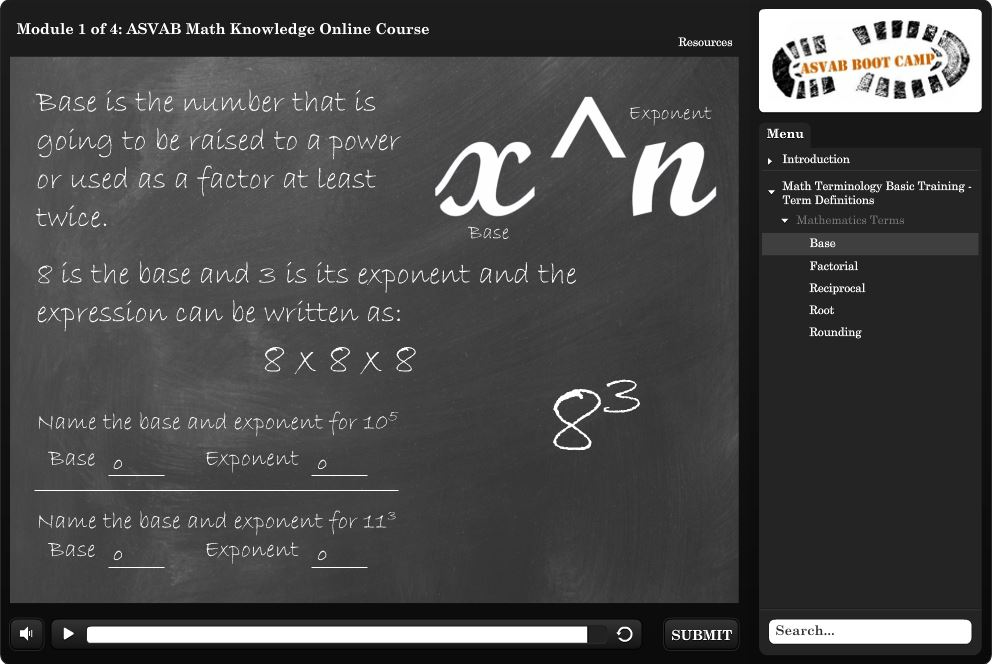# ASVAB Math Practice Online, AnytimeWhen you became interested in joining the military, being tested on your math knowledge was probably something you thought was over the day you completed high school. No more finding the mysterious value of “x”, the square root of a number, or adding, subtracting, and multiplying without a calculator?

As it turns out, you will always need math for something. That’s why you’re faced with the ASVAB Mathematics Knowledge and ASVAB Arithmetic Reasoning test sections.

## Online ASVAB math practice material is easy to access from anywhere and at any time. Before you begin studying, here is a handy list of topics you need to know.

Outline of information to study for the ASVAB Mathematics Knowledge subtest:

• Basic math and algebra terminology
• Solving algebra equations and expressions
• Geometric shapes and their formulas
• Long division/multiplication without a calculator

Outline of information to study for the ASVAB Arithmetic Reasoning subtest:

• Order of operations
• Calculations with fractions, decimals, percentages, ratios and proportions
• Formulas for Shapes
• Factorials
• Number pattern series
• Types of averages: mean, median and mode
• Scale drawings

If you’re a little nervous about starting your ASVAB math practice online, we’re here to set your mind at ease. This isn’t climbing Mount Everest. This is the type of math you already studied in high school. During the test, you’ll be asked to solve some word problems as well as straightforward equation questions.

Example of ASVAB Mathematics Knowledge question:
If a = 2b, then 5 + 7(a – 2b) =

The correct answer is 5. If you substitute 2b for a, the equation is 5 + 7(2b – 2b) = . The two variables cancel out because you multiply the 7 with each 2b first (this is because of the order of operations) and then are left with the correct answer of 5.

Example of ASVAB Arithmetic Reasoning question:
Amanda’s bank account has a balance of \$928.16.  She deposits a check for \$412.83 and then uses her bank card to pay for a \$17.22 lunch, get \$36.59 worth of gas and buy a new pair of shoes for \$67.90.  How much money does she have in the bank account at the end of the day?

The correct answer is \$1,219.28. Solving this question is requires using addition and subtraction. Start with the starting balance, add the deposit, and subtract the expenditures. Here is the starting balance plus the deposit: \$928.16 + \$412.83 = \$1,340.99.  Now add up the total expenditures, which are \$17.22 + \$36.59 + \$67.90 = \$121.71, so now you have the total amount of expenditures. Subtract the expenditures total from the current balance total to get \$1,340.99 – \$121.71 = \$1,219.28.

You Can Start Your ASVAB Math Practice Online Today

This ASVAB Math Study Pack includes online courses and online practice tests that you can access any time, at your convenience. The training courses will prepare you for the Arithmetic Reasoning and Mathematics Knowledge sections of the ASVAB. The ASVAB math practice tests allow you to directly test yourself on new concepts as you learn them. In addition, the ASVAB math practice tests provide the same look and feel as the live computerized test. Each practice test covers all the objectives and utilizes the same content areas as the real test so you know what to expect on test day.

For more detailed information about what to expect on the ASVAB, including test format and timing, visit our About the ASVAB page.